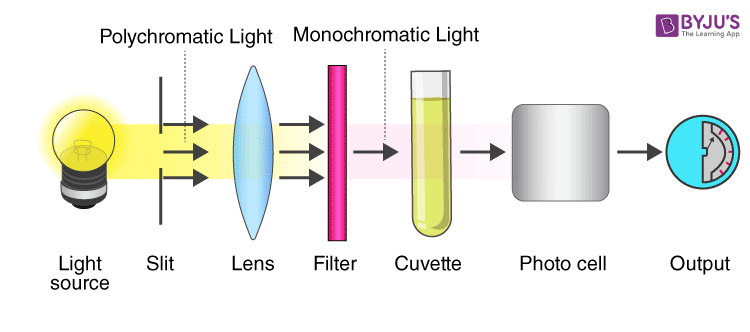Checkout JEE MAINS 2022 Question Paper Analysis : Checkout JEE MAINS 2022 Question Paper Analysis :

# Colorimeter

## What is Colorimeter?

A colorimeter is a device that is used in Colorimetry. It refers to a device which helps specific solutions to absorb a particular wavelength of light. The colorimeter is usually used to measure the concentration of a known solute in a given solution with the help of the Beer-Lambert law. The colorimeter was invented in the year 1870 by Louis J Duboscq.

Check out the derivation of Beer-Lambert law here.

## Principle of Colorimeter

It is a photometric technique which states that when a beam of incident light of intensity Io passes through a solution, the following occur:

• A part of it is reflected which is denoted as Ir
• A part of it is absorbed which is denoted as Ia
• Rest of the light is transmitted and is denoted as It

Therefore, Io = Ir + Ia + It

To determine Ia the measurement of Io and It is sufficient therefore, Ir is eliminated. The amount of light reflected is kept constant to measure Io and It.

Colorimeter is based on two fundamental laws of photometry. We have discussed them below:

### Beer’s law:

According to this law the amount of light absorbed is proportional to the solute concentration present in solution.

Log10 Io/It = asc

where,

as is absorbency index

c is the concentration of solution

### Lambert’s law:

According to this law the amount of light absorbed is proportional to the length as well as thickness of the solution taken for analysis.

A = log10 Io/It = asb

Where,

A is the test absorbance of test

as is the standard absorbance

b is the length / thickness of the solution

## Diagram of Colorimeter## Working of Colorimeter

Step 1: Before starting the experiment it is important to calibrate the colorimeter. It is done by using the standard solutions of the known solute concentration that has to be determined. Fill the standard solutions in the cuvettes and place it in the cuvette holder of colorimeter.

Step 2: A light ray of a certain wavelength, which is specific for the assay is in the direction of the solution. The light passes through a series of different lenses and filters. The coloured light navigates with the help of lenses, and the filter helps to split a beam of light into different wavelengths allowing only the required wavelength to pass through it and reach the cuvette of the standard test solution.

Step 3: When the beam of light reaches’ cuvette, it is transmitted, reflected, and absorbed by the solution. The transmitted ray falls on the photodetector system where it measures the intensity of transmitted light. It converts it into the electrical signals and sends it to the galvanometer.

Step 4: The electrical signals measured by the galvanometer are displayed in the digital form.

Step 5: Formula to determine substance concentration in test solution.

A = ∈cl

For standard and test solutions

∈ and l are constant

AT = CT ….. (i)

AS = CS ….. (ii)

From the above two equations,

AT × CS = AS × CT

CT = (AT/AS) × CS

Where,

CT is the test solution concentration

AT is the absorbance/optical density of test solution

CS is the standard concentration

AS is the absorbance / optical density of standard solution

## Uses of Colorimeter

• It is used in laboratories and hospitals to estimate biochemical samples such as urine, cerebrospinal fluid, plasma, serum, etc.
• It is used in the manufacturing of paints.
• It is used in textile and food industry.
• It is used in the quantitative analysis of proteins, glucose, and other biochemical compounds.
• It is used to test water quality.
• It is used to determine the concentration of haemoglobin in the blood.

Some benefits are as follows:

It is an inexpensive method, widely used in the quantitative analysis of coloured samples, easy to carry, and transport.

Analysis of colourless compounds is not possible, does not work in IR and UV regions.

## Frequently Asked Questions – FAQs

### What is the principle of colorimeter?

Colorimetry is the field in which a coloured compound’s concentration in a solution is measured. A colorimeter, also known as a philtre photometer, is an analytical system that calculates the absorption of a certain wavelength of light as a method to calculate a solution concentration.

### What is difference between spectrophotometer and colorimeter?

In order to have an objective measure of colour characteristics, a colorimeter is typically any instrument that characterizes colour samples. A spectrophotometer is a photometer (an instrument for determining the intensity of light) that can determine the intensity or, more precisely, the wavelength of light as a function of colour.

### What wavelength does a colorimeter use?

By adding another reagent to it, a solution’s colour can be intrinsic or derived. At a user-selectable wavelength, the Colorimeter measures the amount of light reflected through a sample. You can choose from four wavelengths: 430 nm, 470 nm, 565 nm, and 635 nm using the front panel arrow keys.

### What is colorimetry absorbance?

Absorbance is a unit measure of the amount of light that passes through a volume of liquid from a specific wavelength, relative to the maximum possible amount of light available at that wavelength.

### How colorimeter is used in real world?

To track the growth of a bacterial or yeast colony, colorimeters are commonly used. When used for the evaluation of colour in bird plumage, they have accurate and extremely detailed results. They are used in various foods and drinks, including vegetable products and sugar, to quantify and track colour.